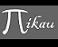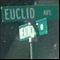# Math Is Fun Forum

Discussion about math, puzzles, games and fun.   Useful symbols: ÷ × ½ √ ∞ ≠ ≤ ≥ ≈ ⇒ ± ∈ Δ θ ∴ ∑ ∫  π  -¹ ² ³ °

You are not logged in.

## #1 2008-09-30 00:58:24

MathsIsFunRegistered: 2005-01-21
Posts: 7,711

### DARPA Mathematical Challenges

DARPA have issued 23 broad mathematical challenges.

Money is available!

Multiple awards are anticipated.  The amount of resources made available to this BAA will depend on the quality of the proposals received and the availability of funds.

The Challenges:

Mathematical Challenge One:  The Mathematics of the Brain
Develop a mathematical theory to build a functional model of the brain that is mathematically consistent and predictive rather than merely biologically inspired.

Mathematical Challenge Two:  The Dynamics of Networks
Develop the high-dimensional mathematics needed to accurately model and predict behavior in large-scale distributed networks that evolve over time occurring in communication, biology and the social sciences.

Mathematical Challenge Three:  Capture and Harness Stochasticity in Nature
Address Mumfords call for new mathematics for the 21st century. Develop methods that capture persistence in stochastic environments.

Mathematical Challenge Four:  21st Century Fluids
Classical fluid dynamics and the Navier-Stokes Equation were extraordinarily successful in obtaining quantitative understanding of shock waves, turbulence and solitons, but new methods are needed to tackle complex fluids such as foams, suspensions, gels and liquid crystals.

Mathematical Challenge Five:  Biological Quantum Field Theory
Quantum and statistical methods have had great success modeling virus evolution. Can such techniques be used to model more complex systems such as bacteria? Can these techniques be used to control pathogen evolution?

Mathematical Challenge Six:  Computational Duality
Duality in mathematics has been a profound tool for theoretical understanding. Can it be extended to develop principled computational techniques where duality and geometry are the basis for novel algorithms?

Mathematical Challenge Seven:  Occams Razor in Many Dimensions
As data collection increases can we do more with less by finding lower bounds for sensing complexity in systems? This is related to questions about entropy maximization algorithms.

Mathematical Challenge Eight:  Beyond Convex Optimization
Can linear algebra be replaced by algebraic geometry in a systematic way?

Mathematical Challenge Nine:  What are the Physical Consequences of Perelmans Proof of Thurstons Geometrization Theorem?
Can profound theoretical advances in understanding three dimensions be applied to construct and manipulate structures across scales to fabricate novel materials?

Mathematical Challenge Ten:  Algorithmic Origami and Biology
Build a stronger mathematical theory for isometric and rigid embedding that can give insight into protein folding.

Mathematical Challenge Eleven:  Optimal Nanostructures
Develop new mathematics for constructing optimal globally symmetric structures by following simple local rules via the process of nanoscale self-assembly.

Mathematical Challenge Twelve:  The Mathematics of Quantum Computing, Algorithms, and Entanglement
In the last century we learned how quantum phenomena shape our world. In the coming century we need to develop the mathematics required to control the quantum world.

Mathematical Challenge Thirteen:  Creating a Game Theory that Scales
What new scalable mathematics is needed to replace the traditional Partial Differential Equations (PDE) approach to differential games?

Mathematical Challenge Fourteen:  An Information Theory for Virus Evolution
Can Shannons theory shed light on this fundamental area of biology?

Mathematical Challenge Fifteen:  The Geometry of Genome Space
What notion of distance is needed to incorporate biological utility?

Mathematical Challenge Sixteen:  What are the Symmetries and Action Principles for Biology?
Extend our understanding of symmetries and action principles in biology along the lines of classical thermodynamics, to include important biological concepts such as robustness, modularity, evolvability and variability.

Mathematical Challenge Seventeen:  Geometric Langlands and Quantum Physics
How does the Langlands program, which originated in number theory and representation theory, explain the fundamental symmetries of physics? And vice versa?

Mathematical Challenge Eighteen:  Arithmetic Langlands, Topology, and Geometry
What is the role of homotopy theory in the classical, geometric, and quantum Langlands programs?

Mathematical Challenge Nineteen:  Settle the Riemann Hypothesis
The Holy Grail of number theory.

Mathematical Challenge Twenty:  Computation at Scale
How can we develop asymptotics for a world with massively many degrees of freedom?

Mathematical Challenge Twenty-one:  Settle the Hodge Conjecture
This conjecture in algebraic geometry is a metaphor for transforming transcendental computations into algebraic ones.

Mathematical Challenge Twenty-two:  Settle the Smooth Poincare Conjecture in Dimension 4
What are the implications for space-time and cosmology? And might the answer unlock the secret of dark energy?

Mathematical Challenge Twenty-three:  What are the Fundamental Laws of Biology?
This question will remain front and center for the next 100 years. DARPA places this challenge last as finding these laws will undoubtedly require the mathematics developed in answering several of the questions listed above.

"The physicists defer only to mathematicians, and the mathematicians defer only to God ..."  - Leon M. Lederman

Offline

## #2 2008-09-30 03:18:51

mikau
MemberRegistered: 2005-08-22
Posts: 1,504

### Re: DARPA Mathematical Challenges

I find it interesting that so many of these challenges involve applying mathematics to biology. It would be cool if mathematicians could supply assistance to the important study of biology as much as possible, since I would assume most biologists are not advanced mathematicians, and that skill may be able to advance our understanding of the subject, or any subject, more than we realize.

A logarithm is just a misspelled algorithm.

Offline

## #3 2008-09-30 06:22:48

Ricky
ModeratorRegistered: 2005-12-04
Posts: 3,791

### Re: DARPA Mathematical Challenges

since I would assume most biologists are not advanced mathematicians

Biologists are typically not mathematicians, but they do work together an awful lot.  A lot of Biology has been involved heavily with both mathematics and computer science.  I did research last summer involving computational chemistry to be used for proteins.  Algebraic Geometry is used heavily in molecular biology, and database theory is also used in gene sequencing.

"In the real world, this would be a problem.  But in mathematics, we can just define a place where this problem doesn't exist.  So we'll go ahead and do that now..."

Offline